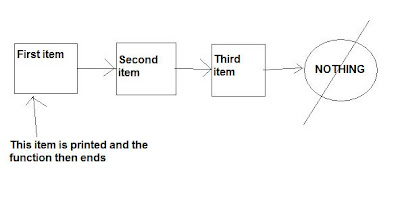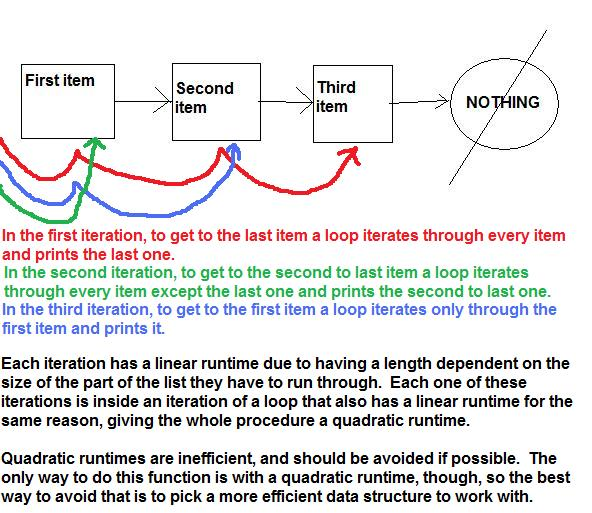## Thursday, September 2, 2010

### Lesson 0. Runtime analysis and Big O notation

Lesson 0. From Constant to Quadratic, a guide to runtime analysis

Welcome to the first tutorial for COMP 15. This tutorial explains runtime analysis (Big O notation). Big O notation is essentially the notation used to measure the runtime of your program, which will be extremely important when you get to the harder projects near the end. One goal of programming is to write code that has as low a big O runtime as possible.

Big O notation looks like this O(n^x), O(1), O(lgn), etc., with n being the size of the data being worked with and x (when applicable) being the power of n. The whole reason why you are learning how to make data structures is to make your program more efficient, and part of that efficiency is runtime.

To really drive home how universal big O notation is, I will not use any code when explaining it. Instead, I will use a generic data structure based on the linked list with pseudocode.

The rules of this data structure are:

1. Each list item points to the next list item, except for the last one, which points to nothing.

2. To access a list item, you must traverse the list, accessing all list items before it.

It looks something like this:So, let’s say we wanted to print out the first list item in the list, and we made a function to do this. This is pseudocode for what the function would do:

printFirstItem(firstItem)

{

print(firstItem);

}

No matter what, the first element is always accessed first, so no matter what, getting to the first item always takes the same amount of time, a CONSTANT amount of time, so the big O notation of the runtime is O(n0), or O(1).Now, let’s say we want to go through EVERY item and print each one out. The pseudocode for that function would be:

printAll(firstItem)

{

go to firstItem;

while(not at end)

{

print(currentItem);

go to nextItem;

}

}

This function traverses the list and accesses every item, and the bigger the list is, the longer the function has to run to get to the end and get the whole list printed, so this function runs at a LINEAR runtime of O(n).Here’s where it starts to get interesting. Let’s make a function that prints the whole list BACKWARDS!

Remember that to access one item, you need to access all previous items. You will see the consequences of that in the pseudocode.

printBack(firstItem)

{

itemToPrint = size;

while(itemToPrint > 0)

{

go to firstItem;
for(int i = 0; i < itemToPrint; i++)
{
go to = nextItem;
}
print(currentItem);
}

}

Wow, that’s a lot of runtime. This means that you have to first of all execute the while loop a number of times equal to the size of the list, which by itself is a process with a O(n) runtime.

But then we have the nested loop! With each iteration of the loop, we have to go through a number of iterations of the for loop ranging from 1 (printing the first item) to the size of the list (printing the last item), which means that the for loop is ALSO dependent on the list’s size, so the for loop has a linear runtime.

Since a for loop with a linear runtime is nested in a while loop with a linear runtime, the number of times the line “go to nextItem;” runs is calculated to n/2(1+n), or .5(n^2 + n). The highest order of the function in big O notation is n^2, and the coefficient ½ is removed, so the big O runtime is linear times linear, or QUADRATIC, giving it a runtime of O(n^2).

Note: If a loop always runs the same number of times regardless of the size of a data structure, that loop’s runtime is constant and shouldn’t be factored into the runtime.

You may have also noticed that because the range of the number of iterations in the for loop ranges from 1 to the size of the list, the overall number of iterations is the sum of an arithmetic sequence, n/2(1+n). Those sequences with that sigma notation that your math teacher tortured you with in high school are back in computer science when dealing with runtimes, so make sure to learn that and geometric sequence sums.Now, back to the quadratic runtime. Beyond this there are cubic runtimes, quartic runtimes, and even EXPONENTIAL runtimes (n^n)! (O_O) Quadratic runtimes are NOT good! If you have a constant runtime, then that’s awesome because it can’t go any faster. If you have a logarithmic runtime (not discussed in this tutorial, but you will see them when learning about the tree data structure), then you will have a sleek and fast program that’s really legit. If you have a linear runtime, then you’ve still got a good runtime on that function. Quadratic runtimes are on the borderline. Anything bigger, and you are looking at a slow program.

The ranking of commonly-seen runtimes from shortest to longest is this:

Good

O(1)

O(lgn)

O(n)

O(nlgn)

Borderline

O(n^2)

O(n^2lgn)

O(n^3)

May have a runtime of millions of years (I’m not making this up!)

O(c^n) c = constant

O(n^n)

With the backwards printing on the list we used, we really had no choice because of how the data structure was designed. One important part of programming is picking a data structure that will minimize runtime. Let’s say we had another data structure which was like the list but you could access it from either the front or back end like this.

Then you have a runtime of O(n) when traversing in either direction. The one drawback is that maintaining the bi-directional property of the list may increase runtime. These trade-offs are what makes it so you have to choose the best data structure for whatever program you are making. No data structure is the universal perfect structure, so we’ve got a lot of them to learn! So what are you waiting for? Let’s go start learning them!

#### 1 comment :

1.The huge pictures can be viewed by clicking on them. There will be a lot of huge pictures in this blog.